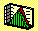2.9 PORTFOLIO STATISTICS

A portfolio of financial securities can be represented by a collection of N numbers, denoted as: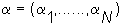where each number ai is the proportion of wealth invested in security i. Sometimes, ai is called the share of security i in the portfolio. The sum of these shares must equal one. Here, we show you how to calculate the expected returns and variance of a portfolio.

If x is a random variable, then so is any multiple of x by some number a. We illustrate this computation for the population mean and population variance of ax: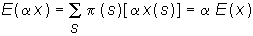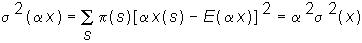Similarly, for some other random variable y, and any number b, then by is also a random variable with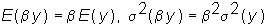.

Finally, for the sum of any two random variables a x + b y, we can calculate the expectation and variance as follows: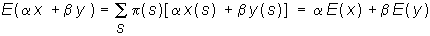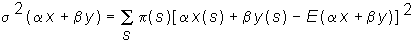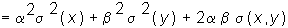These last two formulas are very useful when calculating the expected returns and variances of portfolios.

We describe risk and return characteristics of a portfolio in Summary of Portfolio Statistics, followed by examples.

previous topic

next topic